### The FACTOR Model

The FACTOR modeling language is used for specifying exploratory and confirmatory factor analysis models. You can use other general modeling languages such as LINEQS, LISMOD, PATH, and RAM to specify a factor model. But the FACTOR modeling language is more convenient for specifying factor models and is more specialized in displaying factor-analytic results. For convenience, models specified by the FACTOR modeling language are called FACTOR models.

#### Types of Variables in the FACTOR Model

Each variable in the FACTOR model is either manifest or latent. Manifest variables are those variables that are measured in the research. They must be present in the input data set. Latent variables are not directly observed. Each latent variable in the FACTOR model can be either a factor or an error term.

Factors are unmeasured hypothetical constructs for explaining the covariances among manifest variables, while errors are the unique parts of the manifest variables that are not explained by the (common) factors.

In the FACTOR model, all manifest variables are endogenous, which means that they are predicted from the latent variables. In contrast, all latent variables in the FACTOR model are exogenous, which means that they serve as predictors only.

#### Naming Variables in the FACTOR Model

Manifest variables in the FACTOR model are referenced in the input data set. In the FACTOR model specification, you use their names as they appear in the input data set. Manifest variable names must not be longer than 32 characters. There are no further restrictions on these names beyond those required by the SAS System.

Error variables in the FACTOR model are not named explicitly, although they are assumed in the model. You can name latent factors only in confirmatory FACTOR models. Factor names must not be longer than 32 characters and must be distinguishable from the manifest variable names in the same analysis. You do not need to name factors in exploratory FACTOR models, however. Latent factors named Factor1, Factor2, and so on are generated automatically in exploratory FACTOR models.

#### Model Matrices in the FACTOR Model

Suppose in the FACTOR model that there are p manifest variables and n factors. The FACTOR model matrices are described in the following subsections.

##### Matrix
(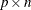) : Factor Loading Matrix

The rows ofrepresent the p manifest variables, while the columns represent the n factors. Each row ofcontains the factor loadings of a variable on all factors in the model.

##### Matrix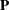() : Factor Covariance Matrix

Thematrix is a symmetric matrix for the variances of and covariances among the n factors.

##### Matrix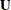(
) : Error Covariance Matrix

Thematrix represents adiagonal matrix for the error variances for the manifest variables. Elements in this matrix are the parts of variances of the manifest variables that are not explained by the common factors. Note that all off-diagonal elements ofare fixed zeros in the FACTOR model.

##### Vector(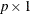) : Intercepts

If the mean structures are analyzed, vectorrepresents the intercepts of the manifest variables.

##### Vector(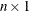) : Factor Means

If the mean structures are analyzed, vectorrepresents the means of the factors.

#### Matrix Representation of the FACTOR Model

Letbe avector of manifest variables,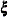be anvector of latent factors, andbe avector of errors. The factor model is written as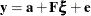With the model matrix definitions in the previous section, the covariance matrix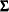() of manifest variables is structured as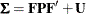The mean vector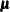() of manifest variables is structured as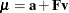#### Exploratory Factor Analysis Models

Traditionally, exploratory factor analysis is applied when the relationships of manifest variables with factors have not been well-established in research. All manifest variables are allowed to have nonzero loadings on the factors in the model. First, factors are extracted and an initial solution is obtained. Then, for ease of interpretation a final factor solution is usually derived by rotating the factor space. Factor-variable relationships are determined by interpreting the final factor solution. This is different from the confirmatory factor analysis in which the factor-variable relationships are prescribed and to be confirmed.

So far, confirmatory and exploratory models are not distinguished in deriving the covariance and mean structures. These two types of models are now distinguished in terms of the required structures or restrictions in model matrices.

In PROC CALIS, the initial exploratory factor solution is obtained from a specific confirmatory factor model with restricted model matrices, which are described as follows:

• The factor loading matrixhas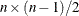fixed zeros at the upper triangle portion of the matrix.

• The factor covariance matrixis an identity matrix, which means that factors are not correlated.

• The error covariance matrixis a diagonal matrix.

• Except for METHOD=FIML or METHOD=LSFIML and robust methods with the ROBUST option, the mean structures are not modeled by default—that is, the intercept vectoror the factor mean vectorare not parameterized in the model.

• With METHOD=FIML or METHOD=LSFIML, the application of the robust methods with the ROBUST option, the use of the MEAN statement, or the specification of the MEANSTR option, the mean structures are modeled. The intercept vectorcontains p free parameters, and the factor mean vectoris a zero vector.

The intercept vectoris parameterized in the FIML method because the first-order moments (that is, the variable means) of the data have to be analyzed with the FIML treatment of the incomplete observations. For robust methods, the observations are reweighted during estimation, so the intercept vectormust also be parameterized to accommodate the use of robust means in computing robust covariances. Estimation that is done using methods other than FIML or without the robust methods usually ignores the analysis of the mean structures because they are saturated and do not affect the fitting of covariance structures.

With the exploratory factor specification, you do not need to specify the patterns of the model matrices. PROC CALIS automatically sets up the correct patterns for the model matrices. For example, for an analysis with nine variables and three factors, the relevant model matrices of an exploratory FACTOR model have the following patterns, where * denotes free parameters in the model matrices: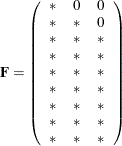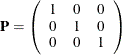and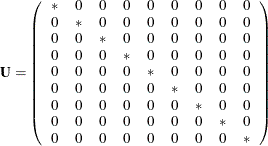If METHOD=FIML or METHOD=LSFIML, the elements of the intercept vectorare all free parameters, as shown in the following: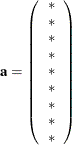The factor mean vectoris a fixed zero vector.

If an initial factor solution is rotated afterward, some of these matrix patterns are changed. In general, rotating a factor solution eliminates the fixed zero pattern in the upper triangle of the factor loading matrix. If you apply an orthogonal rotation, the factor covariance matrixdoes not change. It is an identity matrix before and after rotation. However, if you apply an oblique rotation, in general the rotated factor covariance matrixis not an identity matrix and the off-diagonal elements are not zeros.

The error covariance matrixremains unchanged after rotation. That is, it would still be a diagonal matrix. For the FIML estimation, the rotation does not affect the estimation of the intercept vectorand the fixed factor mean vector.

#### Confirmatory Factor Analysis Models

In confirmatory FACTOR models, there are no imposed patterns on the,,, andmodel matrices. All elements in these model matrices can be specified. However, for model identification, you might need to specify some factor loadings or factor variances as constants.

The only model restriction in confirmatory FACTOR models is placed on, which must be a diagonal matrix, as in exploratory FACTOR models too.

For example, for a confirmatory factor analysis with nine variables and three factors, you might specify the following patterns for the model matrices, where * denotes free parameters in the model matrices: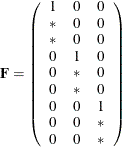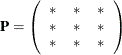andIn this confirmatory factor model, mean structures are not modeled. In addition, there are some distinctive features that underscore the differences between confirmatory and exploratory models:

• Factor loading matrixcontains mostly zero elements and few nonzero free parameters, a pattern which is seen in most confirmatory factor models. In contrast, in exploratory factor models most elements in thematrix are nonzero parameters.

• Factor loading matrixcontains fixed values of ones. These fixed values are used for model identification purposes (that is, identifying the scales of the latent variables). In general, you always have to make sure that your confirmatory factor models are identified by putting fixed values in appropriate parameter locations in the model matrices. However, this is not a concern in exploratory FACTOR models because identification has been ensured by imposing certain patterns on the model matrices.

• The nonzero off-diagonal parameters in the factor covariance matrixindicate that correlated factors are hypothesized in the confirmatory factor model. This cannot be the case with the initial model of exploratory FACTOR models, where thematrix must be an identity matrix before rotation.

#### Summary of Matrices in the FACTOR Model

Let p be the number of manifest variables and n be the number of factors in the FACTOR model. The names, roles, and dimensions of the FACTOR model matrices are shown in the following table.

Matrix

Name

Description

Dimensions_FACTFCOV_

Factor covariance matrix_FACTERRV_

Error covariance matrix_FACTINTE_

Intercepts_FACTMEAN_

Factor means#### Specification of the Exploratory Factor Model

Because all initial model matrices of exploratory FACTOR models are predefined in PROC CALIS, you do not need to specify any other parameters in the model matrices. To obtain desired factor solutions, you can use various options for exploratory factor analysis in the FACTOR statement. These options are the EFA-options in the FACTOR statement. Two main types of EFA-options are shown as follows:

• options for factor extraction: COMPONENT, HEYWOOD, and N=.

• options for factor rotation: GAMMA=, NORM=, RCONVERGE=, RITER=, ROTATE=, and TAU=.

For example, the following statement requests that three factors be extracted, followed by a varimax rotation of the initial factor solution:

factor n=3 rotate=varimax;


See the for details about the EFA-options.

#### Specification of the Confirmatory Factor Model

To specify a confirmatory FACTOR model, you specify the factor-variable relationships in the FACTOR statement, the factor variances and error variances in the PVAR statement, the factor covariances in the COV statement, and the means and intercepts in the MEAN statement.

##### Specification of Factor-Variable Relationships

The CFA-spec in the FACTOR statement is for specifying the factor-variables relationships. For example, in the following statement you specify three factors F1, F2, and F3 that are related to different clusters of observed variables V1V9:

factor
F1  ===> V1-V3  = 1. parm1 (.4) parm2 (.4),
F2  ===> V4-V6  = 1. parm3 parm4,
F3  ===> V7-V9  = 1. parm5 parm6 (.3);


In the specification, variable V1 has a fixed loading of 1.0 on F1. Variables V2 and V3 have loadings on F1 also. These two loadings are free parameters named parm1 and parm2, respectively. Initial estimates can be set in parentheses after the free parameters. For example, both parm1 and parm2 have initial values at 0.4. Similarly, relationships of factor F2 with V4V6 and of factor F3 with V7V9 are defined in the same FACTOR statement. Providing initial estimates for parameters is optional. In this example, parm3, parm4, and parm5 are all free parameters without initial values provided. PROC CALIS can determine appropriate initial estimates for these parameters. See the descriptions of CFA-spec in the for more details about the syntax.

##### Specification of Factor Variances and Error Variances

You can specify the factor variances and error variances in the PVAR statement. For example, consider the following statement:

pvar F1-F3  = fvar1-fvar3,
V1-V9  = evar1-evar9 (9*10.);


In the PVAR statement, you specify the variances of factors F1, F2, and F3 as free parameters fvar1, fvar2, and fvar3, respectively, and the error variances for manifest variables V1V9 as free parameters evar1evar9, respectively. Each of the error variance parameters is given a starting value at 10. See the for more details about the syntax.

##### Specification of Factor Covariances

You can specify the factor covariances in the COV statement. For example, you specify the covariances among factors F1, F2, and F3 in the following statement:

cov F1 F2  = cov12,
F1 F3  = cov13,
F2 F3  = cov23;


The covariance parameters are named cov12, cov13, and cov23, respectively. They represent the lower triangular elements of the factor covariance matrix. See the for more details about the syntax.

##### Specification of Means and Intercepts

If mean structures are of interest, you can also specify the factor means and the intercepts for the manifest variables in the MEAN statement. For example, consider the following statement:

mean F1-F3 = fmean1-fmean3,
V1-V9 = 9*12.;


In this statement, you specify the factor means of F1, F2, and F3 as free parameters fmean1, fmean2, and fmean3, respectively, and the intercepts for variables V1V9 as fixed parameters at 12. See the for more details about the syntax.

##### Naming the Factors

For the exploratory FACTOR model, PROC CALIS generates the names for the factors automatically. For the confirmatory FACTOR model, you can specify the names for the factors. Unlike the LINEQS model, in the confirmatory FACTOR model you do not need to use the 'F' or 'f' prefix to denote factors in the model. You can use any valid SAS variable names for the factors, especially those names that reflect the nature of the factors. To avoid confusions with other names in the model, some general rules are recommended. See the section Naming Variables and Parameters for these general rules about naming variables and parameters.

#### Default Parameters in the FACTOR Model

Default parameters in the FACTOR model are different for exploratory and confirmatory factor models.

For the exploratory FACTOR model, all fixed and free parameters of the model are prescribed. These prescribed parameters include a fixed pattern for the factor loading matrix, a diagonal pattern for the error variance matrix, and an identity matrix for factor covariance matrix. This means that factors are uncorrelated in the estimation. However, if you specify an oblique rotation after the estimation of the factor solution, the factors could become correlated. See the section Exploratory Factor Analysis Models for more details about the patterns of the exploratory FACTOR model. Because all these patterns are prescribed, you cannot override any of these parameters for the exploratory FACTOR model.

For the confirmatory FACTOR model, the set of default free parameters of the confirmatory FACTOR model includes the following:

• the error variances of the observed variables; these correspond to the diagonal elements of the uniqueness matrix• the variances and covariances among the factors; these correspond to all elements of the factor covariance matrix• the intercepts of the observed variables if the mean structures are modeled; these correspond to all elements of the intercept vectorPROC CALIS names the default free parameters with the _Add prefix (or the _a prefix for the case of default intercept parameters infor exploratory factor models), followed by a unique integers for each parameter. Except for the exploratory factor model, you can override the default free parameters by explicitly specifying them as free, constrained, or fixed parameters in the COV, MEAN, or PVAR statement.

In addition to default free parameters, another type of default parameter is the fixed zeros applied to the unspecified parameters in the loading matrixand the factor means in the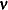vector. Certainly, you use the FACTOR and MEAN specifications to override those default zero loadings or factor means and set them to free, constrained, or fixed parameters. Notice that the uniqueness matrixin the confirmatory factor model is a diagonal element. You cannot specify any of its off-diagonal elements—they are always fixed zeros by the model restriction.